Science, Maths & Technology

### Become an OU studentEveryday maths 2

Start this free course now. Just create an account and sign in. Enrol and complete the course for a free statement of participation or digital badge if available.

# 3 Volume

The volume of a shape is how much space it takes up. You might need to calculate the volume of a space or shape if, for example, you wanted to know how much soil to buy to fill a planting box or how much concrete you need to complete your patio.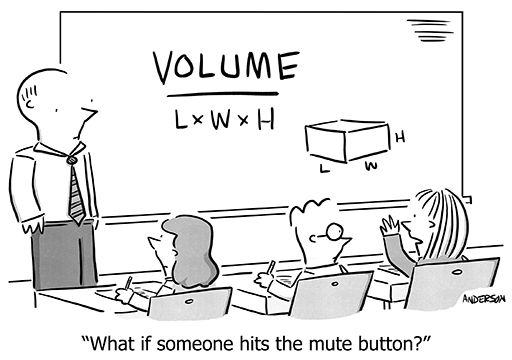Figure _unit5.3.1 Figure 33 A volume cartoon

You’ll need your area skills in order to calculate the volume of a shape. In fact, as you already know how to calculate the area of most shapes, you are one simple step away from being able to find the volume of most shapes too!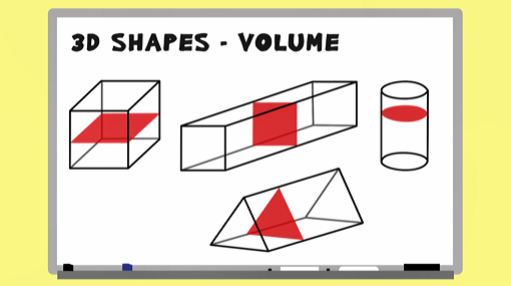Interactive feature not available in single page view (see it in standard view).

## Case study _unit5.3.1 Example: Calculating volume 1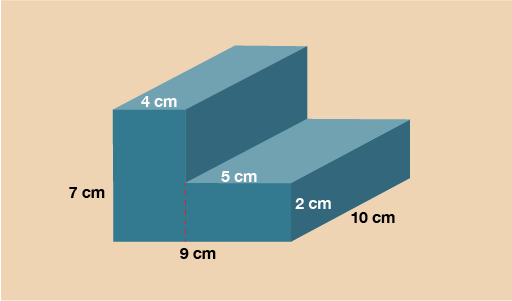Figure _unit5.3.2 Figure 34 Calculating volume 1

The cross section on this shape is the L shape on the front. In order to work out the area you’ll need to split it up into two rectangles as you practised in the previous part of this section.

Splitting vertically:

### Extract _unit5.3.1

Rectangle 1 = 7 × 4 = 28 cm2

Rectangle 2 = 5 × 2 = 10 cm2

Area of cross section = 28 + 10 = 38 cm2

Now you have the area of the cross section, multiply this by the length to calculate the volume.

### Extract _unit5.3.2

V = 38 × 10 = 380 cm3

## Case study _unit5.3.2 Example: Calculating volume 2

The last example to look at is a cylinder. The cross section of this shape is a circle. You’ll need to use the formula to find the area of a circle in the same way you did in the previous part of this section.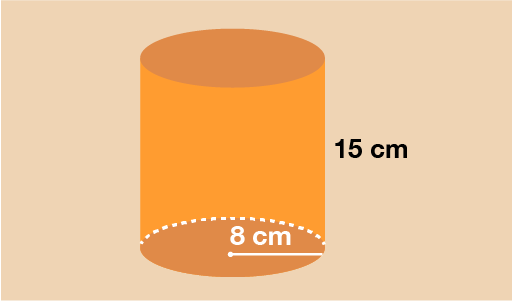Figure _unit5.3.3 Figure 35 Calculating volume 2

You can see that the circular cross section has a radius of 8 cm. To find the area of this circle, use the formula:

### Extract _unit5.3.3

A = πr2

A = 3.142 × 8 × 8

A = 201.088 cm2

Now you have the area, multiply this by the length of the cylinder to calculate the volume.

### Extract _unit5.3.4

V = 201.088 × 15 = 3016.32 cm3

Now try yourself in the activity below.

## Activity _unit5.3.1 Activity 7: Calculating prism volume

Work out the volume of the 3D trapezium (trapezoidal prism) shown below.

### Box _unit5.3.1

Hint: Go back to the section on area and remind yourself of the formula to find the area of a trapezium.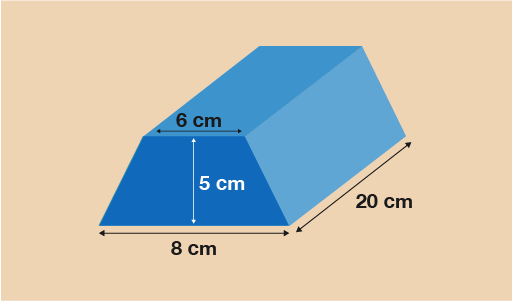Figure _unit5.3.4 Figure 36 Calculating the volume of a prism

The cross section is a trapezium so you will need the formula:

• A =

• A =

• A =

• A =

• A = 35 cm2

Now you have the area of the cross section, multiply it by the length of the prism to calculate the volume.

• V = 35 × 20 = 700 cm3

The final part of this session will look at scale drawings and plans. It will require your previous knowledge of ratio as scale drawings are really just another application of ratio.

## Summary

In this section you have learned:

• that volume is the space inside a 3D shape or space

• how to find the volume of prisms such as cuboids, cylinders and triangular prisms.